Courses

# VLE Algorithms for Low to Moderate Pressures Civil Engineering (CE) Notes | EduRev

## Civil Engineering (CE) : VLE Algorithms for Low to Moderate Pressures Civil Engineering (CE) Notes | EduRev

The document VLE Algorithms for Low to Moderate Pressures Civil Engineering (CE) Notes | EduRev is a part of the Civil Engineering (CE) Course Thermodynamics.
All you need of Civil Engineering (CE) at this link: Civil Engineering (CE)

VLE Algorithms for Low to Moderate Pressures

The next level of complexity in VLE algorithms arise when one has to account for non-ideal behaviour for both the gas and liquid phases. This may obtain at pressures away from atmospheric and if the constituent molecules form a non-ideal liquid phase. The general approach to VLE of such system involves correcting both sides of the Raoult’s law to incorporate the effect of non-ideal behaviour. If the pressures are moderately high the truncated virial EOS may be used to describe the gas phase behaviour, whereas the liquid phase non-ideality is defined by a suitable activity coefficient model. The activity coefficient based approach is preferred for moderate pressures, as under such conditions the liquid phase properties may be conveniently regarded as independent of pressure, hence only temperature effects on the activity coefficients need be accounted for. This approach, of course, is rendered inaccurate at relatively high pressures, where both the gas and liquid phases need to be described using fugacity coefficients derived typically from a cubic (or a higher order) EOS. This is dealt with in the next section. Presently the VLE algorithms for low to moderate pressure range are introduced.  The starting point is the eqn. (6.126):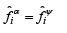..(6.126)
Applying it to VLE: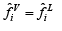..(6.127)
For gas phase, we use eqn. 6.129: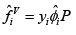For liquid phase (using eqn. 6.164):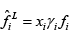Applying eqn. 6.127: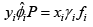..(7.45)

From basic fugacity function for liquid phase (eqn.6.119):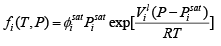..(6.119)

Using eqn. 6.119 in 6.128 we may write the phase equilibria relation as: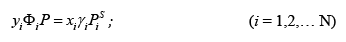..(7.46)

Where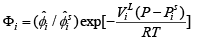..(7.47)

One may show that the Pontying (exponential factor) in the last equation is usually ~ 1 for low to moderate pressure range, hence one may write: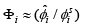..(7.48)

For a gas mixture obeying the truncated virial EOS (by eqn. 6.98):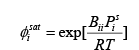..(7.49)

Specifically for a binary using eqns. 6.149 and 6.150: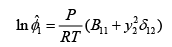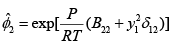..(6.149)

Using the last four equations it follows that:                                                 ..(6.150)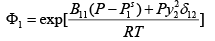..(7.50)

And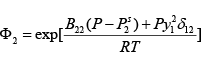..(7.51)

It may be shown that for a multi-component the general expression for Φi is provided by: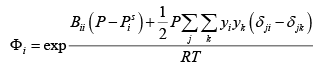..(7.52)
Now,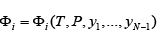And: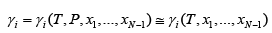..(7.53)

The approximation made in eqn. 7.53 is a reasonable one, as at low to moderate pressures the dependence of γi γi on ‘P’ may be neglected (as at such conditions the liquid phase properties are not strongly pressure dependent). The same five classes as provided in table 7.1 may be solved using this modified form of the Raoult’s law. In all cases eqn. 7.46 provides the starting point for calculation, which may be re-written is two principal alternate forms as follows: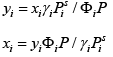..(7. 54 & 7.55)

Since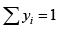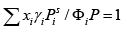Or: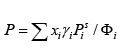........(7.56)

Similarly since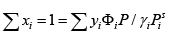it follows that: '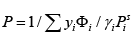........(7.57)

We may also re-write eqn. 7.46 in terms of the K-factor (as used for Raoult’s Law in eqn. 7.37) as follows: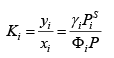........(7.58)

Accordingly:

y= Kixi                                                                                               ..(7.59)

Or: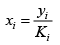........(7.60)

From eqn. 7.59, it follows that: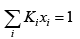........(7.61)

From eqn. 7.60: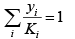Note that when Φi= γi = eqn. 7.46 reduces to the ideal case of Raoult’s Law.

Bubble pressure:

Given T and {xi } , to calculate P and { yi} :

a) Start with given T, { xi } , Antoine constants, ∈ (error value for convergence)

b) Set all {Φi} = 1.0, Evaluate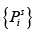, {γi } , Calculate P using eqn. 7.56

c) Calculate { yi } using eqn.7.54

d) Now evaluate {Φi} , using eqns. 7.52

e) Calculate Pnew using eqn. 7.56

f) Is δP <∈ ?

g) If ‘No’, go to step ‘c’ and calculate new { yi } with last {Φi}

h) If ‘Yes’, end at last P, and { yi}

Dew Point Pressure:

Given T and {yi } , to calculate P and {xi}

a) Start with T and {yi } ; Antoine constants; ε and δ (error values for convergence); start with Raoult’s law by setting all {Φi} = 1.0, and all {γi} = 1.0; Evaluate, then calculate P using eqn. 7.57; Now evaluate { xi } by eqn. 7.55; Evaluate {γi } using appropriate activity coefficient model Liquid-phase; recalculate  P using eqn. (7.65),  revise {Φ i} using given { yi } and last P. b) Calculate new set { xi } using eqn. 7.55

b) Calculate new set { xi } using eqn. 7.55

c) Normalize { xi } using i (n)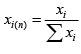, and use normalized { xi } to compute {γi }

d) Use last {γi} to calculate P by eqn. 7.57 e) Is δP <ε ?

f) If ‘Yes’ then Plast =Pd(f)

Bubble Temperature:

Given P and {xi } , to calculate T and {yi }

a) Solve for T and {yi } first by assuming Raoult’s Law algorithm for bubble temperature

b) Using solution in ‘a’ estimate {Ki } using eqn. 7.58 with the given values of P and {xi } ; latest values of T and {yi }

c) Next calculate {Kixi }

d) Calculate all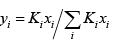e) Using normalized { yi } , recalculate {Ki } and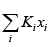f) Haschanged? If yes return to step ‘d’

g) Ifhas not changed between two successive iterations between steps ‘c’ and ‘d’ is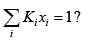If yes, the last values of T and { yi ≡ Kixi } give the final bubble temperature Tb(f) ,and vapour compositions.

i) If no, and last> 1 then Tlast >Tb(f) ; revise to new T as: Tnew  = Tlast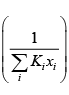and return to step (c).and return to step ‘b’.

j) If no, and last ii<1 then Tlast >Tb(f) ; revise to new T as: new last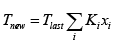and return to step (c).and return to step ‘b’

Dew Temperature:

Given P and { yi  } , to calculate T and {xi  }

a) Solve for T and {xi  } first by assuming Raoult’s Law algorithm for dew temperature

b) Using solution in ‘a’ estimate {Ki  } using eqn. 7.58 with the given values of P and { yi  } ; latest values of T and {xi }

c) Next calculate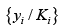d) Calculate all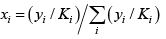e) Using normalized { xi } , recalculate {Ki } and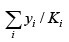f) Haschanged? If yes return to step ‘d’

g) Ifhas not changed between two successive iterations between steps ‘c’ and ‘d’ ish) If yes, the last values of T and { xi ≡ yi /Ki } give the final dew temperature Td(f) ,and liquid phase compositions.

i) If no, and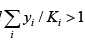, then Tlast <Td( f) ; revise to new T as: new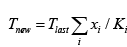and return to
step (b).

j) If no, and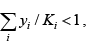, then Tlast >Td ( f) ; revise to new T as: newand return to
step (b).

Flash Distillation Calculations : The procedure for non-ideal systems takes a form similar to that adopted for systems obeying Raoult’s Law except that one needs to additionally check for existence of both liquid and vapour phases following flash. The algorithm comprises the following steps.

b) At the given T, calculate dew pressure Pd by putting { y} = { z }

c) Next calculate bubble pressure Pb by putting { x } = {z }

d) Is P< P<Pb ? If no, the vapour phase has not formed.

e) If yes, compute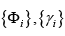, and V as =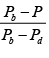f) Use, to get {ki} using eqn.  7.58

g) Then use eqn. 7.42 and 7.43 to evaluateϕ and dϕ / dV .

h) Using Newton-Raphson method, findV

i) With last V compute { xi } using eqn. 7.40 and { yi } by eqn. 7.38

j) Re-calculate, and {ki}  using eq n. 7.58 ii i Φ γ K

k) Check if the change in each parameter xi ,yi , and V between steps ‘e’ and ‘j’ is within predefined error values chosen for convergence.

l) If yes, then the last values of xi ,yi , and V constitute the solution

m) If no, return to step ‘f’ with the last values of xi ,yi , and V

Offer running on EduRev: Apply code STAYHOME200 to get INR 200 off on our premium plan EduRev Infinity!

## Thermodynamics

56 videos|92 docs|33 tests

,

,

,

,

,

,

,

,

,

,

,

,

,

,

,

,

,

,

,

,

,

;